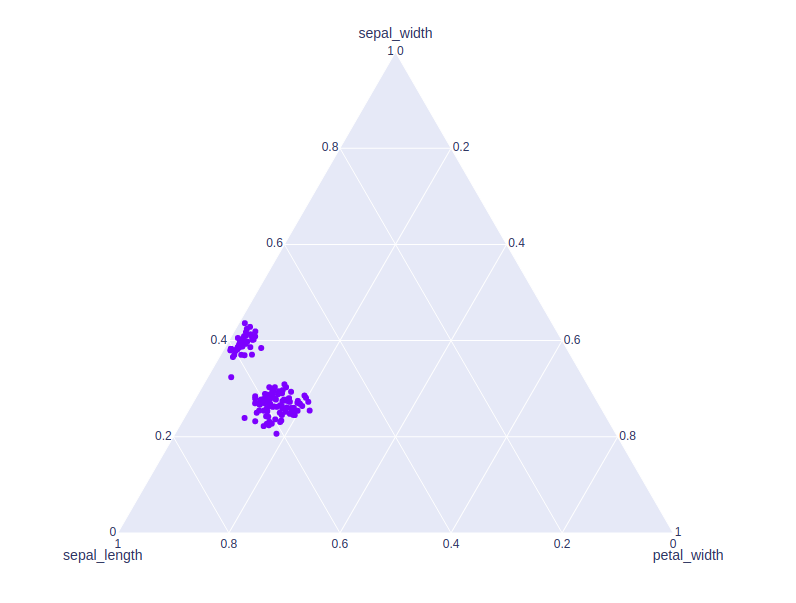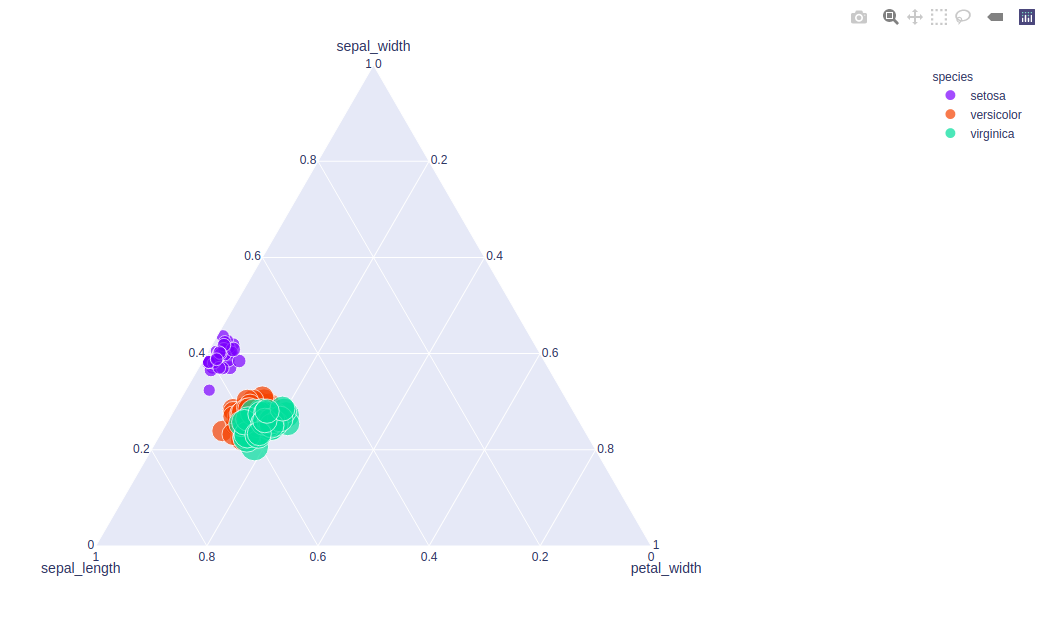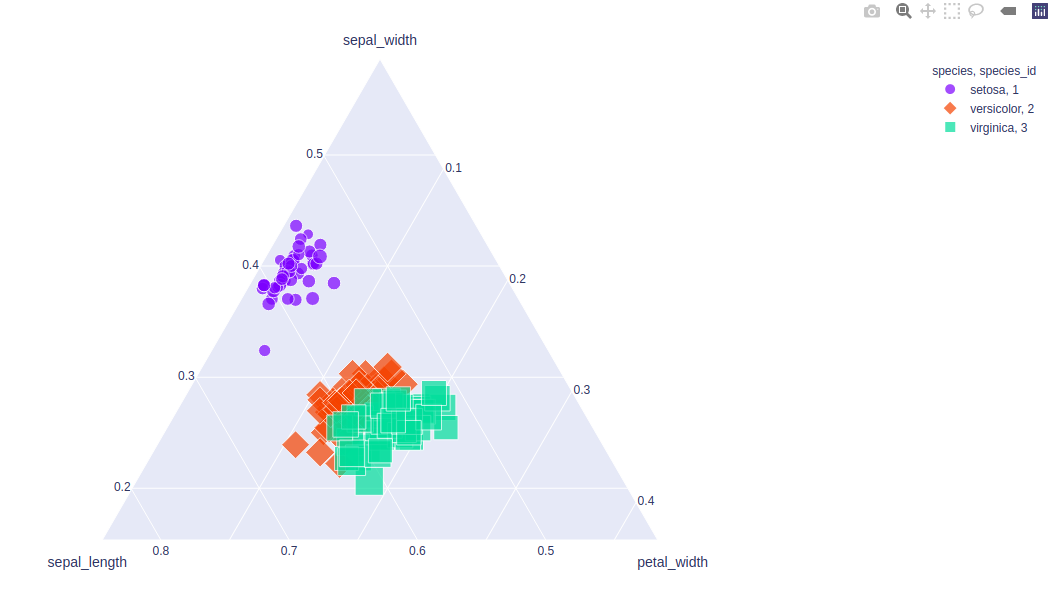# plotly.express.scatter_ternary() function in Python

• Last Updated : 17 Jul, 2020

Plotly library of Python can be very useful for data visualization and understanding the data simply and easily. Plotly graph objects are a high-level interface to plotly which are easy to use.

## plotly.express.scatter_ternary() function

This method is used to create a ternary plot. A ternary plot is used to depics the ratio of three variables on an equilateral triangle.

Attention geek! Strengthen your foundations with the Python Programming Foundation Course and learn the basics.

To begin with, your interview preparations Enhance your Data Structures concepts with the Python DS Course. And to begin with your Machine Learning Journey, join the Machine Learning - Basic Level Course

Syntax: plotly.express.scatter_ternary(data_frame=None, a=None, b=None, c=None, color=None, symbol=None, size=None, text=None, hover_name=None, hover_data=None, size_max=None, title=None, template=None, width=None, height=None)

Parameters:

data_frame: DataFrame or array-like or dict needs to be passed for column names.

a, b, c:  These parameters are either a name of a column in data_frame, or a pandas Series or array_like object. These are used to position marks along the a, b, and c axis in ternary coordinates respectively.

color: This parameters assign color to marks.

symbol: This parameter is used to assign symbols to marks. It is either a name of a column in data_frame, or a pandas Series or array_like object.

size: This parameter is used to assign mark sizes. It is either a name of a column in data_frame, or a pandas Series or array_like object.

hover_name:  Values from this column or array_like appear in bold in the hover tooltip.

hover_data: This parameter is used to appear in the hover tooltip or tuples with a bool or formatting string as first element, and list-like data to appear in hover as second element Values from these columns appear as extra data in the hover tooltip.

title: The title of the figure.

width: Set the width of the figure.

height: Set the height of the figure.

Example 1:

## Python3

 `import` `plotly.express as px`` ` `df ``=` `px.data.iris()`` ` `plot ``=` `px.scatter_ternary(df, a ``=` `'sepal_width'``,``                          ``b ``=` `'sepal_length'``, ``                          ``c ``=` `'petal_width'``)``plot.show()`

Output:Example 2: Using the size and color arguments

## Python3

 `import` `plotly.express as px`` ` `df ``=` `px.data.iris()`` ` `plot ``=` `px.scatter_ternary(df, a ``=` `'sepal_width'``, ``                          ``b ``=` `'sepal_length'``,``                          ``c``=``'petal_width'``,``                          ``color ``=` `'species'``,``                          ``size ``=` `'petal_length'``)``plot.show()`

Output:Example 3: Using the symbol parameter

## Python3

 `import` `plotly.express as px`` ` `df ``=` `px.data.iris()`` ` `plot ``=` `px.scatter_ternary(df, a ``=` `'sepal_width'``,``                          ``b ``=` `'sepal_length'``, ``                          ``c ``=` `'petal_width'``, ``                          ``color ``=` `'species'``,``                          ``size ``=` `'petal_length'``,``                          ``symbol ``=` `'species_id'``)``plot.show()`

Output:My Personal Notes arrow_drop_up